Clutch Prep is now a part of Pearson
Ch.14 - Chemical EquilibriumWorksheetSee all chapters

# Chemical Equilibrium

See all sections
Sections
Chemical Equilibrium
ICE Chart
Le Chatelier's Principle
The Reaction Quotient
Equilibrium Expressions

Most chemical reactions never go to completion where all the reactants are converted into products. Instead these reactions reach a chemical equilibrium

###### Chemistry & Equilibrium

Concept #1: Understanding Chemical Equilibrium.

Transcript

Hey guys! In this brand new video, we're going to take a look at the Equilibrium State and figure out when exactly do some reactions reach equilibrium.
Now first we're going to say that most chemical reactions never go to completion. When we say the word completion all that really means is that our reactants do not completely convert into products. Our reaction that goes to completion, every amount of reactant that's there gets broken down in order to build up to make our products. But we're going to say that this doesn't happen with all chemical reactions. So there's going to be some amount of reactant left. Now that amount could be a very small amount or could be a very large amount. It all depends on the chemical reaction. Now since our reactant amount is always going to be some amount, we're going to say reaction concentrations do not go down to 0. Now we're going to say instead these chemical reactions reach a state of chemical equilibrium in which the reaction moves in the forward and the reverse direction. Remember we talked about this, we said that these reactions have double arrows, one going in the forward one going in the reverse. And we're going to say because they can go in the forward and the reverse direction, they're called reversible reactions.
Here we have a typical reaction, we have reactant A going in the forward direction to give us product B. And just realize we're going in the forward direction here with this forward facing arrow and we use K1 to signify this forward direction. Now on the same way the reaction could go in the opposite direction where some of our product actually breaks down itself in order to rebuilt some of the reactant that was lost. When we’re going in the reverse direction we use K-1. The minus signifies that we're going in the reverse direction. Now if we're going to look at this plot here, you can see on this plot that our blue line represents our reactant A, our red line represents our product B. And what you should take from this chart is this, we're going to be losing reactants, so we lose reactants to make products. So that's the most common thing that we have to take from this image. And what you should realize here is eventually product amount will stop increasing and reactant amount will stop decreasing and they'll reach a plateau for both of them. So there amounts were going to be held constant after a while. It's at this 5 minute mark where they become constant in their concentrations. So we're going to say equilibrium happens at that exact moment. So that's how we're able to tell a reaction has reached chemical equilibrium. The reactant and the product concentrations or amounts have been held constant. Now reactions are still going on on a molecular level but for every amount of reactant we lose, it's okay because our products are going in their reverse direction to make up for what we just lost. So in that way their levels maintain a same number overtime. So we're going to say once our amounts plateau that's when equilibrium is reached.
Now knowing that, I want you guys to take a look at this practice question on the bottom and try your best to answer it on your own. Once you figured out the answer, come back on our next video and click on the explanation button after answering and see if your answer matches up with my own. Good luck on this question guys!

A reaction reaches equilibrium once the rate of the forward reaction equals the rate of the reverse reaction. Once at equilibrium there is no net change in the concentration of reactants or products.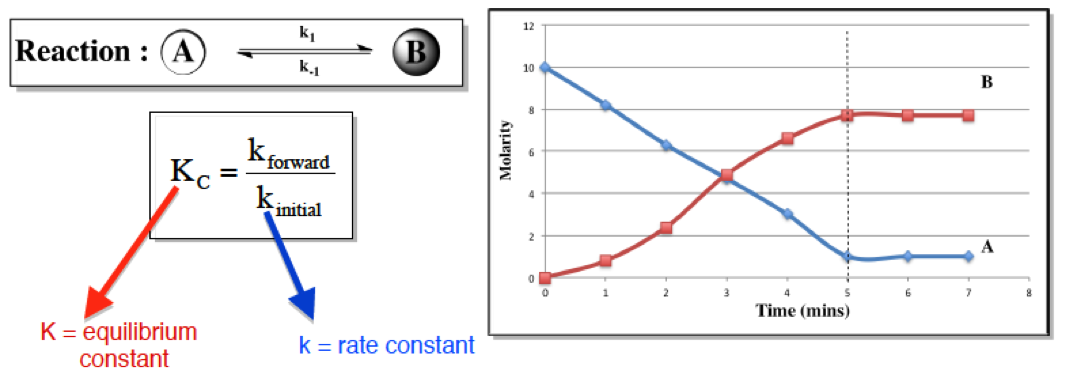Whereas the rate constant, k, is affected by factors dealing with the rate of reaction, the equilibrium constant, K, is affected by only temperature.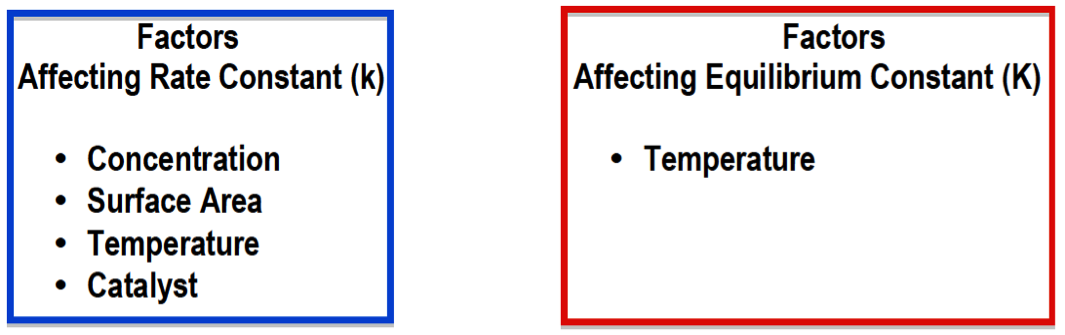Practice: Which one of the following statements does not describe the equilibrium state?

a. While at equilibrium, a dynamic process is still occurring.

b. The concentration of the reactants is equal to the concentration of the products.

c. The concentration of the reactants and products reach a constant level.

d. At equilibrium, the net concentration of all species is not changing.

e. All are true.

###### The Equilibrium Constant K

Associated with any reaction at equilibrium is the equilibrium constant K. Its numerical value determines if reactants or products are more greatly favored within a reaction.

Concept #2: Understanding the Equilibrium Constant.

Transcript

Hey guys! In this brand new video, we're going to take a look at the Equilibrium Constant.
Now we're going to say that the variable we use to illustrate our equilibrium constant is the variable K. And we're going to say K is a number equal to the ratio of products to reactants at a given temperature. Why do I say at a given temperature? Because we're going to say that temperature directly affects our equilibrium constant K. Increasing the temperature will increase our K value, decreasing our temperature will decrease our K value. Now we're going to say it's a ratio of products to reactants so we're going to say that here K = products/reactants. Now we're going to say that K is important because its magnitude tells us how far to the left or to the right our chemical reaction is at a given temperature. So we're going to say if K is greater than 1 then products are favored over reactants. And think about it, this makes sense because we said K = products/reactants so let's say our products are 10 and our reactants are 1, K = 10 definitely greater than 1. We're going to say when products are favored over our reactants that mean we're making more products. How do we make more products? By going in the forward direction. So the forward direction would be favored. Now in the opposite way, if K is less than 1, so let's say we have in this case products are 1 but our reactants are 10, we'd have a K less than 1. So in this case, if K is less than 1 then reactants are favored over products. Which means our reaction is heading in the reverse direction. So the reverse direction would be favored. So just remember these 2 differences, when K is greater than 1, when K is less than 1. Now what if we say K was equal to 1? We know that this is products/reactants so that means our products and reactants would have to be equal, let's say they're both 10. 10/10 is 1. So when K = 1 we're going to say both our reactant and our product amounts are equal to one another. Now we're going to say the equilibrium constant K takes into account all the stats of matter except 2, it doesn't look at solids and it doesn't look at liquids, it ignores those 2 states for matter.

The equilibrium constant K is a ratio of products to reactants. It only deals with gaseous or aqueous compounds.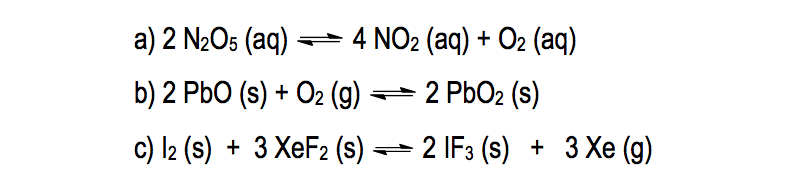Example #1: Write the equilibrium expression for the following reaction:

Transcript

So it looks at it only cares about really gases and also aqueous. Aqueous just means that the solvent is water, so a compound is dissolved in water. It would be aqueous.
Now knowing this, let's take a look at the following Example. It says:
Write the equilibrium expression for the following reaction.
Here we have 2 and 2 N2O5 (aq) gives us 4 NO2 (aq) + O2 (aq). It's important to look at the phases because remember if it's a solid or a liquid we ignore it. Now we're going to say here K = products/reactants. So we're going to have our products as NO2. And remember you have to use the coefficients in these calculations. The number in front of NO2 is a 4, so that 4 will become the power, so it's going to be (NO2)4 * O2, O2. just has a coefficient of 1 in front of it which we don't have to show, divide it by N2O5, again the coefficient in front of N2O5 is 2 so that becomes a power.

K = products = (NO2)4 * (O2)
reactants (N2O5)2

So we would say that this represents our equilibrium expression or our equilibrium equation. Same thing, equilibrium expression, equilibrium equation.
Now that we've seen this one, let's look at B. For B we're going to say here, we're going to ignore this compound because it's a solid and we're going to ignore this compound because it's a solid. Here we're going to say K = products/, like before. But here's the thing, we're going to say we don't have any products available but you have to put something on top. We're going to say it's equal to 1. Solids and liquids are ignored and in place of them we're going to put 1. We're going to say this because things such as pressured don't really affect solids and liquids like they do gases and aqueous compounds, that's why they're equal to 1 because their number’s being held constant. So we're going to replace solids and liquids with the number 1. And on the bottom we'd have O2.

K = products = 1
reactants (O2)1

Now different way your professor could give you this is, we know there's a 1 here so this could also be or O2 inverse 1. So be aware of both type of answers. Both are correct, both are saying the same thing. Inverse 1 just means 1 over whatever it is.

K = products = 1 or O2-1
reactants (O2)1

For the next one, again we ignore solids. The only thing that we don't ignore is this gas. So here K = products/reactants, so it just equal Xe3 because of the 3 over 1. But we don’t need to put the 1 because anything over 1 is the same exact thing. So that would be our equilibrium expression.
K = (Xe)3
So A was pretty simple but remember the difference is in B and C, we ignore the solids and the liquids. Now that you've seen this, I want you guys to attempt to answer question that follows this one, the practice 1. Here I want you to tell me who's greater in amount, is it products or is it reactants? From that you have to remember what do we say about K? When it's greater than 1 who's favored? When it's less than 1 who's favored? When it's equal to 1 who's favored? Remembering that would be a great way for you to approach this problem. Good luck guys!

Practice: State which is greater in amount: reactants or products, based on the given equilibrium constant, K.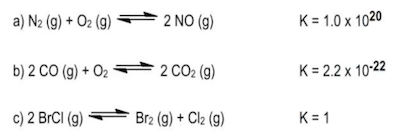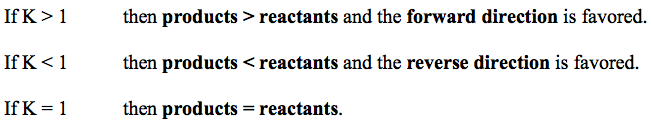Practice: The decomposition of nitrogen monoxide can be achieved under high temperatures to create the products of nitrogen and oxygen gas.

6 NO(aq) ⇌ 3 N2(aq) + 3 O2(aq)

a) What is the equilibrium equation for the reaction above?

b) What is the equilibrium expression for the reverse reaction.

Practice: The equilibrium constant, K, for 2 NO (g) + O2 (g) ⇌ 2 NO2 (g) is 6.9 x 102

What is the [NO] in an equilibrium mixture of gaseous NO, O2, and NO2 at 500 K that contains 1.5 x 10 –2 M O2 and 4.3 x 10 –3 M NO2?

###### Kp and Kc

When dealing with equilibrium units in terms of pressure the equilibrium constant of Kp must be used, but if the equilibrium units are in molarity then we must use the equilibrium constant of Kc

Concept #3: The Different Types of Equilibrium Constants.

Transcript

Hey guys in this new video we're going to take a look at different types of the Equilibrium Constants.
Now one thing that we're going to realize by going through Chemistry 1 and Chemistry 2 is that "K" is a very very popular variable. We have it everywhere. We have a rate constant K. We have actually a K for acids and bases, we call them Ka and Kb. Ka is called our acid association constant, whereas Kb is called our bases association constant. Now we're talking about different types of Equilibrium Constants. So "K" is really really popular when it comes to calculations in Chemistry.
Now, we've talked about the equilibrium constant up to this point. we said that it's equal to products over reactants. And we should realize this that we have different types of Equilibrium Constants based on the units that we're using. Now we're going to say when dealing with gases we usually use the equilibrium constant Kp. We're going to say Kp uses the partial pressure unit of atmospheres.
And were going to say that when dealing with aqueous solutes, remember aqueous means that water is dissolving bicompound. So when we're using aqueous solutes, we tend to use the equilibrium constant Kc. Now Kc uses the concentration unit of molarity and remember molarity is moles over liters. And also remember molarity is the same thing as concentration and the same thing as solubility.
So, we've seen that we have Kp and Kc now. And we're going to say that these two are related together by this equation. Kp = Kc (RT) ∆n. Here we're going to say that R is called our Gas Constant and it equals 0.08206 liters times atmospheres over moles times K. Now remember there's another R variable out there it’s 8.314 joules over moles times K. But we only use that one when we're dealing with energy, velocity or speed. In this case we are not dealing with energy, velocity or speed. We are dealing with Equilibrium Concentrations. So that's where we're using this particular R. We're going to say that T represents our Temperature in Kelvin. And there we're going to say most importantly is our ∆n exponent. ∆n equals moles of gas as products minus moles of gas. Again remember gas as reactants. So we've started out with the broad idea of an Equilibrium Constant K but now we've delve than deeper to Kp and Kc.

Kp and Kc are related to one another by the following equation below: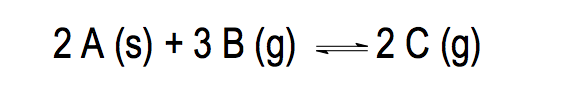Example #2: For the reaction above, the Kc = 4.9 x 10-9 at 25oC. Which of the following statements is true?

1. The reaction is favored in the forward direction.
2. The concentration of the products is greater than the concentration of the reactants.
3. The reaction is favored in the reverse direction.
4. The value of Kp will be larger than the value of Kc

Practice: Methane (CH4) reacts with hydrogen sulfide to yield hydrogen gas and carbon disulfide, a solvent used in the manufacturing rayon and cellophane. What is the value of Kc at 1000 K if the partial pressures in an equilibrium mixture at 1000 K are 0.20 atm methane, 0.15 atm hydrogen sulfide, 0.30 atm carbon disulfide and 0.10 atm hydrogen gas?

CH4 (g) + 2 H2S (g) ⇌ 4 H2 (g) + CS2 (g)

Determination of the value for Δn can help us determine if Kp is greater than, less than or equal to Kc

Practice: In which of the given reactants is Kp greater than, less than and equal to Kc?

a) SO3 (g) + NO (g) ⇌ SO2 (g) + NO2 (g)

b) P4 (s) + 5 O4 (g) ⇌ P4O10 (s)

c) 4 NH3 (g) + 3 O2 (g) ⇌ 2 N2 (g) + 6 H2O (g)

Practice: Given the hypothetical reaction 2 A (s) + ? B (g) ⇌ 3 C (g), Kp = 0.0105 and Kc = 0.45 at 250 degrees Celsius. What is the value of the coefficient of B?

###### Hess’s Law and the Equilibrium Constant K

The old concept of Hess’s Law can be applied to our new concept of the Equilibrium Constant K.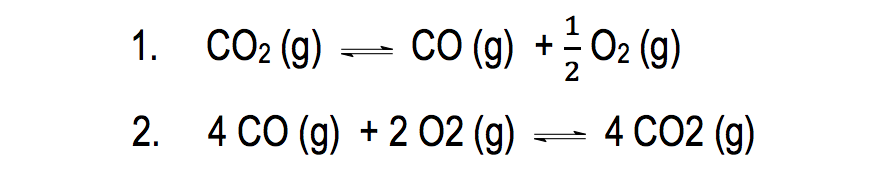Example #3: The equilibrium constant K for the first reaction is 6.83 x 10-12 at 1000 K. Calculate K for the second reaction.

Transcript

Hey guys, in this new video, we’re going to take a look at Hess’s Law at least kind of. Now, we’re going to say that when it comes to Hess’s Law, we learned that the total enthalpy change, so total ΔH of a reaction by taking into account each individual step, now we we’re going to do the same exact thing except instead of using ΔH, we’re going to use our equilibrium constant K. So pay very close attention to what I do here for these two particular examples.
EXAMPLE 1: The equilibrium constant K for the reaction
CO2 (g) ⇄ CO (g) + ½ O2 (g)
is 6.83 x 10-12 at 1000 K. Calculate for the reaction
4 CO (g) + 2 O2 (g) ⇄ 4 CO2 (g)
Now, I’m asking you to find a new K for this new reaction. Now, what you should realize here is that both of these reactions are the same. They both have CO2, they both have CO, and they both have O2. They’re the same exact equation. The only difference here is I changed and manipulated the one on the top so that it now looks like this one. So what we need to figure out is what changes that I do and what effect will that have on the equilibrium constant K.
So we’re going to say first, CO2 was a reactant but now it’s a product. These guys CO (g) + ½ O2 (g) are a product and now they’re a reactant 4 CO (g) + 2 O2 (g). So it looks like I reversed the reaction.
Just realize that when I reverse the reaction, K becomes the reciprocal, so K with is 6.83 x 10-12 well now it becomes 1/6.83 x 10-12. It becomes the reciprocal of whatever it was. Now, that’s one change that I did.
The other change that I did, it looks like I multiplied by 4 because here this CO2 has a 4 now.
CO2 (g) ⇄ CO (g) + ½ O2 (g)
4 CO (g) + 2 O2 (g) ⇄ 4 CO2 (g)
This CO has a 4 now
CO2 (g) ⇄ CO (g) + ½ O2 (g)
4 CO (g) + 2 O2 (g) ⇄ 4 CO2 (g)
and when we do 4 x ½ the 2 cancels out with this 4 and that’s how we go this 2 here.
4 CO (g) + 2 O2 (g) ⇄ 4 CO2 (g)
Now, when you multiply by a number, that number becomes the power. So this is going to become to the 4th. So it’s going to be 1/6.83 x 10-12 to the 4.
KC = (1/6.83 x 10-12)4
KC = (1.46 x 1011)4
KC = 4.60 x 1044
So when you multiply by a number, it becomes a power. So what we’re going to do here is just solve for what’s inside first, (1/6.83 x 10-12) that gives us (1.46 x 1011)4, so that’s going to give me 4.60 x 1044. So that will be our answer here.
Remember if you reverse the reaction, then we’re going to say that that becomes the reciprocal 1 over whatever, when we multiply by a number, that’s going to become the power. You multiply by 5, it becomes power of 5.
Let’s say we divide it by a number. Let’s say we divide it by 3. Well, just remember, if you’re dividing by 3, that is the same as multiplying by 1/3 and if you’re multiplying by 1/3, then 1/3 becomes the power.
Let’s say you have to divide by 2. If you’re dividing by 2, that’s the same thing as multiply by ½ and in that way half becomes the power. Okay, so that’s how we get around the dividing aspect of this.

Reversing a reaction gives us the reciprocal of K, while multiplying a reaction by a value makes it the power for K.

Example #4: Calculate the rate constant, Kc, for the reaction below:

H (g) + Br (g) ----> HBr (g)

Use the following information to calculate Kc.

H2 (g) ---> 2 H (g)                                         Kc = 11.8

Br2 (g) ---> 2 Br (g)                                      Kc = 1.15 x 10-5

H2 (g) + Br2 (g) --->  2 HBr (g)                   Kc = 2.78 x 103

Transcript

So here, this one’s a lot more complicated. What we’re going to say here is we need to find the KC value for this equation here.
EXAMPLE 2: Calculate the rate constant, KC, for the reaction below:
H (g) + Br (g) ---> HBr (g) [KC4]
Use the following information to calculate KC.
H2 (g) ---> 2 H (g) KC1 = 11.8
Br2 (g) ---> 2 Br (g) KC2 =1.15 x 10-5
H2 (g) + Br2 (g) ---> 2 HBr (g) KC3 = 2.78 x 103
So our best strategy for questions like this is since we’re trying to isolate that equation above, we have to look and see where do we see each one of these elements.
H (g) + Br (g) ---> HBr (g) [KC4]
So what we need to do first is find H (g) and we're going to notice that we see H (g) right here.
H2 (g) ---> 2 H (g) KC1 = 11.8
Now, we have to manipulate this one in red so it matches up with this one in blue.
H (g) + Br (g) ---> HBr (g) [KC4]
H2 (g) ---> 2 H (g) KC1 = 11.8
So what two changes do I need to do to it? First, the H in red is a product but we need it to be a reactant, so we need to reverse the reaction. Once we reverse it, we also need to realize that there’s a 2 in front of this H, but over here in the blue box, there isn’t a 2, so we need to divide by 2 and we just said dividing by 2 is the same thing as multiplying by half. So we have to reverse it and multiply by a ½.
So now our equation becomes:
H (g) ---> ½ H2 (g)
When we reverse it, K becomes 1/11.8 and because we multiplied by ½, half becomes the power and just remember half power really means square root.
H (g) ---> ½ H2 (g) KC1 = (1/11.8)1/2 = √1/11.8
We're going to say that this right here got manipulated so we no longer care about it.
H2 (g) ---> 2 H (g) KC1 = 11.8
Remember, we did all of this so that the red H (g) that we had matches up with the H (g) in the blue box. We’re basically trying to manipulate these 3 equations so that they look like the compounds in the blue boxes above.
Next, we’re going to see where do we see Br (g).
H (g) + Br (g) ---> HBr (g) [KC4]
Here in the blue box it’s a reactant but over here it’s a product.
Br2 (g) ---> 2 Br (g) KC2 =1.15 x 10-5
Also here in the blue box, it doesn’t have a coefficient in front of it, but here it has a 2. So the two changes are the same, we need to reverse it so it becomes a reactant to and we need to divide by 2 which is the same thing as multiplying by ½. So this becomes:
Br (g) ---> ½ Br2 (g) KC2 =(1/1.15 x 10-5)1/2 = √(1/1.15 x 10-5)
Finally HBr, where do we see HBr? Luckily, we see HBr only one place. We see it right here as a product so they’re both products so that’s good. So there’s no need to reverse, but there’s a 2 in front of the HBr, so what do we need to do. We need to divide by 2 or same thing as multiplied by ½ .
H (g) + Br (g) ---> HBr (g) [KC4]
H2 (g) + Br2 (g) ---> 2 HBr (g) KC3 = 2.78 x 103
So this is going to become:
½ H2 (g) + ½ Br2 (g) ---> HBr KC3 = (2.78 x 103)1/2 = √2.78 x 103
What we’re going to do next is we’re going to cross out intermediate, things that look alike but is a product in one equation and a reactant in another. So we’re going to say here:
H (g) ---> ½ H2 (g)
Product
½ H2 (g) + ½ Br2 (g) ---> HBr
Reactant
So they’re going to cancel out.
Br (g) ---> ½ Br2 (g)
Product
½ H2 (g) + ½ Br2 (g) ---> HBr
Reactant
They cancel out. Whatever doesn’t get cancelled out comes down. So what didn’t get cancelled out?
H (g) + Br (g) ----> HBr (g)
The equation we just isolated matches up with the equation above. Now, all we have to do is we’re going to say KC4 = KC1 x KC2 x KC3. So basically all we have to do here is we have to multiply each one of these right here.
KC4 = (√1/11.8) x (√1/1.15 x 10-5) x (√2.78 x 103)
KC4 = 4526.19
Hope you guys were able to follow along as we did this problem. Just realize, the equation with the missing KC value is the one we have to look at and we just look compound by compound, look to see where do we find them in the given equations below. When we’re able to isolate them, we will just multiply all the K values together and that will give us the overall K value for this equation we’re looking for in the first place.
So just remember the tips that we did. If you reverse it, K becomes the reciprocal. If you multiply it by a number, that becomes the power. If you divide by a number, then it becomes 1 over that number as a power. So if you divide it by 5, it would be multiplying by 1/5. If you divide it by 4, it would be like multiplying by ¼. Those would become the powers. Just remember those small, little principles, you’ll be able to get these questions correct.

Example #5: Hess's Law & Equilibrium Constant K

Example #6: Hess's Law & Equilibrium Constant K